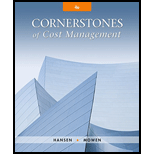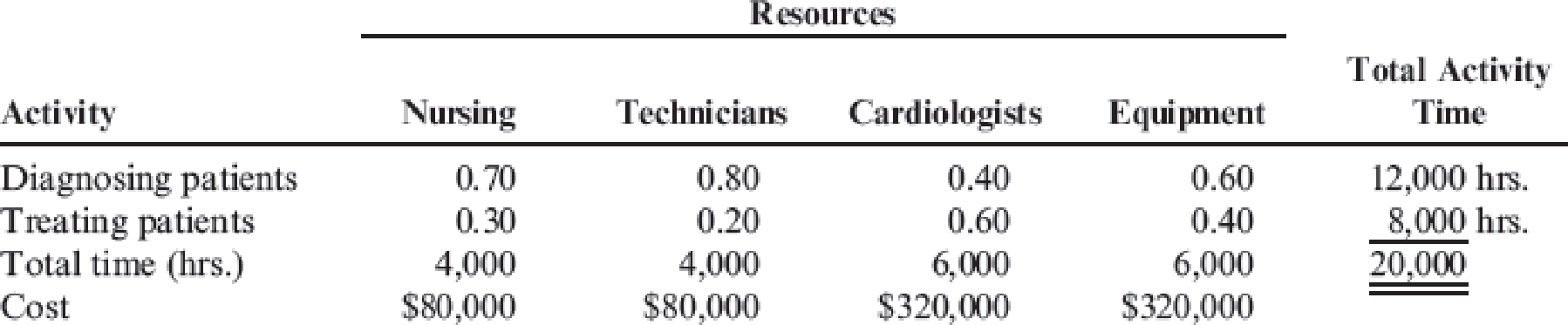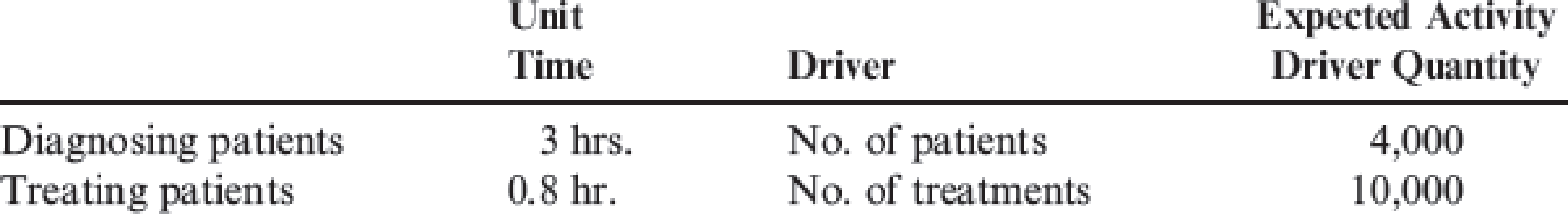# The Bienestar Cardiology Clinic has two major activities: diagnostic and treatment. The two activities use four resources: nursing, medical technicians, cardiologists, and equipment. Detailed interviews have provided the work distribution matrix shown below. The total time estimated corresponds to practical capacity (interviewers adjusted the total time to about 80 percent of the available time). The equipment time is measured in machine hours. Thus, the total time (at practical capacity) in the system is 20,000 hours. In considering the implementation of a TDABC model, the following unit times and transaction information are also provided: Required: 1. Calculate the cost of each activity using the indicated values of the resource drivers. 2. Calculate the capacity cost rate for TDABC. Using the capacity cost rate, calculate the cost of each activity under TDABC. Compare these values with those obtained in Requirement 1 and discuss possible reasons for any differences. 3. Suppose that the actual activity driver quantities are 3,500 and 9,000. Calculate the cost of unused capacity. 4. Suppose that the clinic acquires new equipment that reduces the total time required for the two activities from 6,000 to 4,000 hours. The equipment cost remains the same. Explain how the ABC system would be updated and then describe how TDABC would provide updates. 5. Suppose that diagnosing patients without any cardiac disease takes two hours while diagnosing patients with mildly diseased hearts takes an additional 1.5 hours and those with more severe problems takes an additional two hours. Prepare a time equation and, using the capacity cost rate from Requirement 2, calculate the activity rate for each of the three types of patients.### Cornerstones of Cost Management (C...

4th Edition
Don R. Hansen + 1 other
Publisher: Cengage Learning
ISBN: 9781305970663

#### Solutions

Chapter
Section### Cornerstones of Cost Management (C...

4th Edition
Don R. Hansen + 1 other
Publisher: Cengage Learning
ISBN: 9781305970663
Chapter 4, Problem 35P
Textbook Problem
9 views

## The Bienestar Cardiology Clinic has two major activities: diagnostic and treatment. The two activities use four resources: nursing, medical technicians, cardiologists, and equipment. Detailed interviews have provided the work distribution matrix shown below.The total time estimated corresponds to practical capacity (interviewers adjusted the total time to about 80 percent of the available time). The equipment time is measured in machine hours. Thus, the total time (at practical capacity) in the system is 20,000 hours. In considering the implementation of a TDABC model, the following unit times and transaction information are also provided:Required: 1. Calculate the cost of each activity using the indicated values of the resource drivers. 2. Calculate the capacity cost rate for TDABC. Using the capacity cost rate, calculate the cost of each activity under TDABC. Compare these values with those obtained in Requirement 1 and discuss possible reasons for any differences. 3. Suppose that the actual activity driver quantities are 3,500 and 9,000. Calculate the cost of unused capacity. 4. Suppose that the clinic acquires new equipment that reduces the total time required for the two activities from 6,000 to 4,000 hours. The equipment cost remains the same. Explain how the ABC system would be updated and then describe how TDABC would provide updates. 5. Suppose that diagnosing patients without any cardiac disease takes two hours while diagnosing patients with mildly diseased hearts takes an additional 1.5 hours and those with more severe problems takes an additional two hours. Prepare a time equation and, using the capacity cost rate from Requirement 2, calculate the activity rate for each of the three types of patients.

1.

To determine

Compute the cost of each activity by using the values that are indicated for resource drivers of B Clinic.

### Explanation of Solution

Capacity cost rate: A capacity cost is a cost incurred by a company to enhance their business capacity and operations above a certain level. Capacity cost rate is calculated using the following formula.

Capacity cost rate=Total activity costTotal acticity time Activity cost= Capacity cost rate×Unit time per activity×Number of patients=$40×3×4,000=$480,000

Compute the cost of diagnosing activity by using the indicated values of resource drivers.

 Resource Cost ($) Consumption ratio Diagnosing ($) (a) (b) (a) × (b) Nursing 80,000

2.

To determine

Compute the capacity cost rate of B Clinic for all activities.

3.

To determine

Compute the cost of unused capacity if the actual activity driver quantities are 3,500 and 9,000.

4.

To determine

Assume the clinic acquires new equipment that can reduce total time for the two activities from 6,000 to 4,000 hours and if equipment cost remained the same, describe the manner in which activity based costing would be updated and explain how time driven activity based costing can provide the updates.

5.

To determine

Construct a time equation and compute the activity rate for reach type of patients using the capacity cost rate assuming diagnosing patients without any cardiac disease takes two hours while diagnosing patients with mildly diseased heart attacks takes an additional 1.5 hours and those with more severe problems takes additional two hours.

### Still sussing out bartleby?

Check out a sample textbook solution.

See a sample solution

#### The Solution to Your Study Problems

Bartleby provides explanations to thousands of textbook problems written by our experts, many with advanced degrees!

Get Started

Find more solutions based on key concepts
What does the invisible hand of the marketplace do?

Essentials of Economics (MindTap Course List)

The word persuasion turns some people off. What negative connotations can it have?

Essentials of Business Communication (MindTap Course List)

REQUIRED RATE OF RETURN Suppose rRF = 9%, rM = 14% and bi = 13. a. What is ri, the required rate of return on S...

Fundamentals of Financial Management, Concise Edition (with Thomson ONE - Business School Edition, 1 term (6 months) Printed Access Card) (MindTap Course List)

What does TELOS stand for? How is it helpful?

Accounting Information Systems

Why should policymakers think about incentives?

Principles of Macroeconomics (MindTap Course List)

Why do economists make assumptions?

Principles of Microeconomics (MindTap Course List)

How does an increase in productivity affect business?

Foundations of Business (MindTap Course List)